# Modular curve

(diff) ← Older revision | Latest revision (diff) | Newer revision → (diff)
Jump to: navigation, search

A complete algebraic curve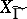uniformized by a subgroupof finite index in the modular group; more precisely, a modular curve is a complete algebraic curve obtained from a quotient space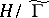, whereis the upper half-plane, together with a finite number of parabolic points (the equivalence classes relative toof the rational points of the boundary of). The best known examples of subgroups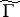of finite index inare the congruence subgroups containing a principal congruence subgroup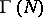of levelfor some integer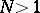, represented by the matrices(see Modular group). The least suchis called the level of the subgroup. In particular, the subgroup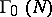represented by matrices which are congruentto upper-triangular matrices has level. Corresponding to each subgroupof finite index there is a covering of the modular curve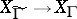, which ramifies only over the images of the points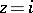,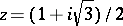,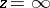. For a congruence subgroupthe ramification of this covering allows one to determine the genus ofand to prove the existence of subgroupsof finite index inwhich are not congruence subgroups (see , Vol. 2, ). The genus of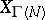isforand equalsa prime number, for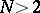. A modular curve is always defined over an algebraic number field (usually overor a cyclic extension of it). The rational functions on a modular curve lift to modular functions (of a higher level) and form a field; the automorphisms of this field have been studied (see ). A holomorphic differential form on a modular curveis given onby a differential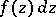(whereis a holomorphic function) which is invariant under the transformationsof; hereis a cusp form of weight 2 relative to. The zeta-function of a modular curve is a product of the Mellin transforms (cf. Mellin transform) of modular forms and, consequently, has a meromorphic continuation and satisfies a functional equation. This fact serves as the point of departure for the Langlands–Weil theory on the relationship between modular forms and Dirichlet series (see , ). In particular, there is a hypothesis that each elliptic curve over(with conductor) can be uniformized by modular functions of level. The homology of a modular curve is connected with modular symbols, which allows one to investigate the arithmetic of the values of the zeta-function of a modular curve in the centre of the critical strip and to construct the-adic zeta-function of a modular curve (see ).

A modular curve parametrizes a family of elliptic curves, being their moduli variety (see , Vol. 2). In particular, for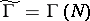a pointofis in one-to-one correspondence with a pair consisting of an elliptic curve(analytically equivalent to a complex torus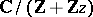) and a point of orderon(the image of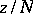).

Over each modular curvethere is a natural algebraic fibre bundle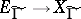of elliptic curves ifdoes not contain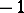, compactified by degenerate curves above the parabolic points of. Powers, where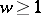is an integer, are called Kuga varieties (see , ). The zeta- functions ofare related to the Mellin transforms of modular forms, and their homology to the periods of modular forms (see , ).

The rational points on a modular curve correspond to elliptic curves having rational points of finite order (or rational subgroups of points); their description (see ) made it possible to solve the problem of determining the possible torsion subgroups of elliptic curves over.

The investigation of the geometry and arithmetic of modular curves is based on the use of groups of automorphisms of the projective limit of the curveswith respect to decreasing, which (in essence) coincides with the groupover the ringof rational adèles. On each modular curvethis gives a non-trivial ring of correspondences(a Hecke ring), which has applications in the theory of modular forms (cf. Modular form, ).